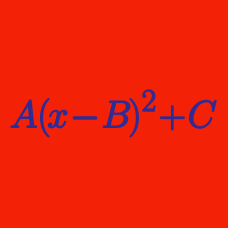Algebra

# Completing the Square - Multiple Variables

For real numbers $$x$$, $$y$$ and $$z$$, function $f(x,y,z)=4x-x^2-y^2-z^2+18$ has a maximum value $$d$$ at $$x=a$$, $$y=b$$ and $$z=c$$. What is the value of $$a+b+c+d$$?

For real numbers $$x$$, $$y$$ and $$z$$, function $f(x,y,z)=x^2+y^2+z^2-4x-4y-4z+14$ has a minimum value $$d$$ at $$x=a$$, $$y=b$$ and $$z=c$$. What is the value of $$a+b+c+d$$?

For real numbers $$x$$ and $$y$$, function $f(x,y)=x^2+2y^2-4x-16y+21$ has a minimum value $$c$$ at $$x=a$$ and $$y=b$$. What is the value of $$a+b-c$$?

For real numbers $$x$$ and $$y$$, function $f(x,y)=2x-x^2+2y-y^2+28$ has a maximum value $$c$$ at $$x=a$$ and $$y=b$$. What is the value of $$a+b+c$$?

What is the maximum value of $$f(f(x))$$ in the domain $$2 \leq x \leq 5$$ for the function $f(x)=x^2-6x+4?$

×